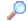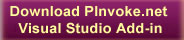SearchDirectory

Desktop Functions:

Smart Device Functions:GlossaryShow Recent ChangesSubscribe (RSS)Misc. Pages
SendInput (user32)

Summary

#### C# Signature:

[DllImport("user32.dll")]
static extern uint SendInput(uint nInputs, INPUT [] pInputs, int cbSize);

#### User-Defined Types:

[StructLayout(LayoutKind.Sequential)]
struct MOUSEINPUT
{
int dx;
int dy;
int mouseData;
int dwFlags;
int time;
IntPtr dwExtraInfo;
}

[StructLayout(LayoutKind.Sequential)]
struct KEYBDINPUT
{
short wVk;
short wScan;
int dwFlags;
int time;
IntPtr dwExtraInfo;
}

[StructLayout(LayoutKind.Sequential)]
struct HARDWAREINPUT
{
int uMsg;
short wParamL;
short wParamH;
}

[StructLayout(LayoutKind.Explicit)]
struct INPUT
{
[FieldOffset(0)] int type;
[FieldOffset(4)] MOUSEINPUT mi;
[FieldOffset(4)] KEYBDINPUT ki;
[FieldOffset(4)] HARDWAREINPUT hi;
}

None.

#### Sample Code:

class Test
{
public enum VK : ushort
{
SHIFT        = 0x10,
CONTROL          = 0x11,
ESCAPE           = 0x1B,
BACK         = 0x08,
TAB          = 0x09,
RETURN           = 0x0D,
PRIOR        = 0x21,
NEXT         = 0x22,
END          = 0x23,
HOME         = 0x24,
LEFT         = 0x25,
UP           = 0x26,
RIGHT        = 0x27,
DOWN         = 0x28,
SELECT           = 0x29,
PRINT        = 0x2A,
EXECUTE          = 0x2B,
SNAPSHOT         = 0x2C,
INSERT           = 0x2D,
DELETE           = 0x2E,
HELP         = 0x2F,
MULTIPLY         = 0x6A,
SEPARATOR        = 0x6C,
SUBTRACT         = 0x6D,
DECIMAL          = 0x6E,
DIVIDE           = 0x6F,
F1           = 0x70,
F2           = 0x71,
F3           = 0x72,
F4           = 0x73,
F5           = 0x74,
F6           = 0x75,
F7           = 0x76,
F8           = 0x77,
F9           = 0x78,
F10          = 0x79,
F11          = 0x7A,
F12          = 0x7B,
OEM_1        = 0xBA,   // ',:' for US
OEM_PLUS         = 0xBB,   // '+' any country
OEM_COMMA        = 0xBC,   // ',' any country
OEM_MINUS        = 0xBD,   // '-' any country
OEM_PERIOD       = 0xBE,   // '.' any country
OEM_2        = 0xBF,   // '/?' for US
OEM_3        = 0xC0,   // '`~' for US
MEDIA_NEXT_TRACK     = 0xB0,
MEDIA_PREV_TRACK     = 0xB1,
MEDIA_STOP           = 0xB2,
MEDIA_PLAY_PAUSE     = 0xB3,
LWIN       =0x5B,
RWIN       =0x5C
}

public struct KEYBDINPUT
{
public WORD wVk;
public WORD wScan;
public DWORD dwFlags;
public LONG time;
public DWORD dwExtraInfo;
};
[StructLayout(LayoutKind.Explicit,Size=28)]
public struct INPUT
{
[FieldOffset(0)] public DWORD type;
[FieldOffset(4)] public KEYBDINPUT ki;
};

void Test()
{
INPUT structInput;
structInput = new INPUT();
structInput.type = Win32Consts.INPUT_KEYBOARD;

// Key down shift, ctrl, and/or alt
structInput.ki.wScan = 0;
structInput.ki.time = 0;
structInput.ki.dwFlags = 0;
structInput.ki.dwExtraInfo = Win32.GetMessageExtraInfo();
if (Shift)
{
structInput.ki.wVk = (ushort)VK.SHIFT;
intReturn = Win32.SendInput(1, ref structInput, (UInt32)sizeof(INPUT));
}
if (Ctrl)
{
structInput.ki.wVk = (ushort)VK.CONTROL;
intReturn = Win32.SendInput(1, ref structInput, (UInt32)sizeof(INPUT));
}
if (Alt)
{
intReturn = Win32.SendInput(1, ref structInput, (UInt32)sizeof(INPUT));
}
if (Win)
{
structInput.ki.wVk = (ushort)VK.LWIN;
intReturn = Win32.SendInput(1, ref structInput, (UInt32)sizeof(INPUT));
}
// Key down the actual key-code
structInput.ki.wVk = vk;
intReturn = Win32.SendInput(1, ref structInput, (UInt32)sizeof(INPUT));
// Key up the actual key-code
structInput.ki.dwFlags = Win32Consts.KEYEVENTF_KEYUP;
intReturn = Win32.SendInput(1, ref structInput, (UInt32)sizeof(INPUT));
// Key up shift, ctrl, and/or alt
if (Shift)
{
structInput.ki.wVk = (ushort)VK.SHIFT;
intReturn = Win32.SendInput(1, ref structInput, (UInt32)sizeof(INPUT));
}
if (Ctrl)
{
structInput.ki.wVk = (ushort)VK.CONTROL;
intReturn = Win32.SendInput(1, ref structInput, (UInt32)sizeof(INPUT));
}
if (Alt)
{
intReturn = Win32.SendInput(1, ref structInput, (UInt32)sizeof(INPUT));
}
if (Win)
{
structInput.ki.wVk = (ushort)VK.LWIN;
intReturn = Win32.SendInput(1, ref structInput, (UInt32)sizeof(INPUT));
}
}
}

#### Alternative Managed API:

Do you know one? Please contribute it!

Documentation
SendInput on MSDN

Do you have...

• helpful tips or sample code to share for using this API in managed code?
• corrections to the existing content?
• variations of the signature you want to share?
• additional languages you want to include?

Select "Edit This Page" on the right hand toolbar and edit it! Or add new pages containing supporting types needed for this API (structures, delegates, and more).Access PInvoke.net directly from VS:Terms of UseFind ReferencesShow Printable VersionRevisions Show changes